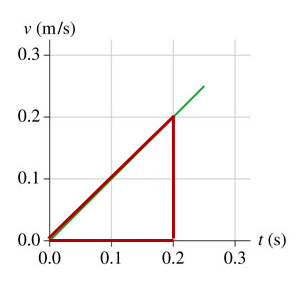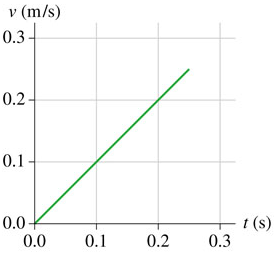# Problem: Scallops eject water from their shells to provide a thrust force. (Figure 1) shows a smoothed graph of actual data for the initial motion of a 25g scallop speeding up to escape a predator.What is the magnitude of the net force needed to achieve this motion?Express your answer to two significant figures and include the appropriate units.

###### FREE Expert Solution

Acceleration,

$\overline{){\mathbf{a}}{\mathbf{=}}\frac{\mathbf{∆}\mathbf{v}}{\mathbf{∆}\mathbf{t}}}$

Newton's second law,

$\overline{){\mathbf{\Sigma F}}{\mathbf{=}}{\mathbit{m}}{\mathbit{a}}}$80% (342 ratings)###### Problem Details

Scallops eject water from their shells to provide a thrust force. (Figure 1) shows a smoothed graph of actual data for the initial motion of a 25g scallop speeding up to escape a predator.What is the magnitude of the net force needed to achieve this motion?

Express your answer to two significant figures and include the appropriate units.